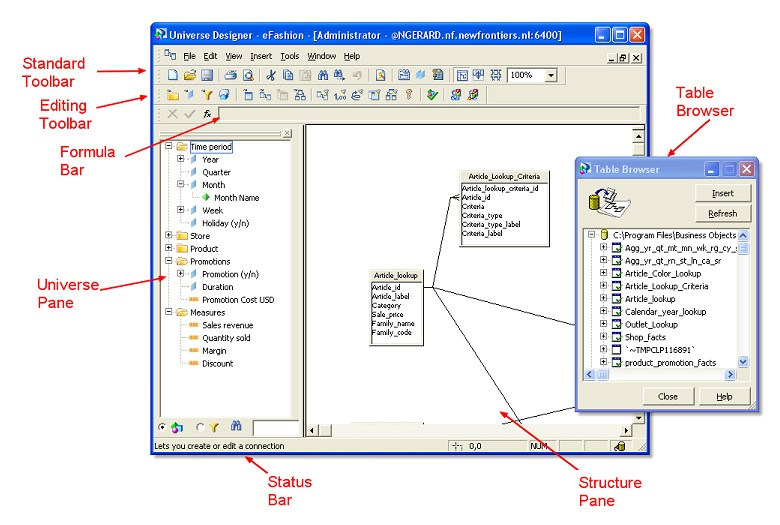# BOBJ - Derived tables

Derived tables are similar to database views, with the advantage that the SQL for a derived table can include prompts.

Derived tables appear in your Designer schema in exactly the same way as normal database tables, but the workflow for creating them is different.

A derived table is defined by an SQL query at the SAP BOBJ - Universe level that can be used as a logical table in Designer.

Derived tables have the following advantages:

• Reduced amount of data returned to the document for analysis.

You can include complex calculations and functions in a derived table. These operations are performed before the result set is returned to a document, which saves time and reduces the need for complex analysis of large amounts of data at the report level.

Derived tables can, in some cases, replace statistical tables that hold results for complex calculations that are incorporated into the universe using aggregate awareness. These aggregate tables are costly to maintain and refresh frequently. Derived tables can return the same data and provide real time data analysis.

## Example

### Creating a derived table to return server information

In this example you want to create objects that allow the user to add information about the database server to their reports.

You create two objects, servername and version, that return the values of the in-built variables @@SERVERNAME and @VERSION in a universe running on an Oracle Server database.

You do this as follows:

• Select Derived Tables on the Insert menu. The Derived Tables dialog box appears.
• Type serverinfo in the Table Name box.
• Creating a schema with tables and joins Using derived tables
• Type in the SQL box. the SQL :
````Select @@SERVERNAME as servername, @@VERSION as version from dual`
```

You must provide aliases in the SQL for all derived columns. Designer uses these aliases to name the columns of the derived tables.

• Click OK.

The derived table serverinfo appears in the Designer schema.

• Create a class called Server Info and add two dimension objects beneath the class, based on the servername and version columns of the serverinfo derived and columns of the table.

Note that the serverinfo table appears in the list of tables like any ordinary database table, and its columns appear in the list of columns like ordinary table columns.

The user can now place the servername and version objects on a report.

### Showing the number of regions in each country

In this example you create a table that shows the number of regions in each country. The SQL is as follows:

``````select country,
count (r.region_id) as number_of_regions
from country c,
region r
where r.country_id = c.country_id
group by country```
```

It is important in this case to alias the column that contains the calculation. Designer uses these aliases as the column names in the derived table. In this case the table has two columns: country and number_of_regions.

## Nested derived tables

A nested derived table (also known as a 'derived table on a derived table') is a table that is derived from at least one existing derived table.

Use the @DerivedTable function to reference a derived table in your nested derived table.

Syntax :

````@DerivedTable(Derived_table_name)`
```

This function is included in the functions catalog in the Derived Tables editor.

Discover More